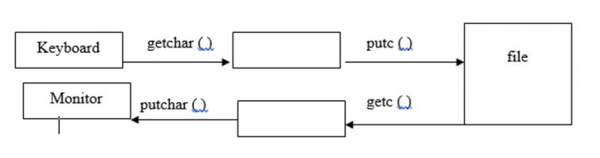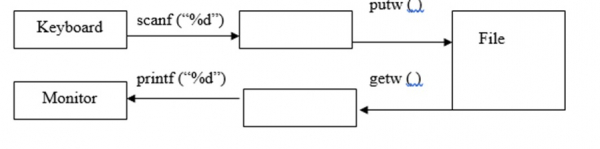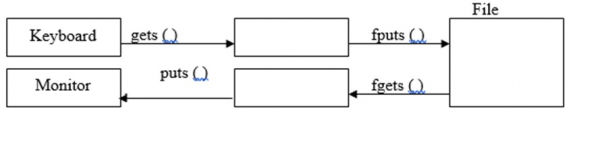# What are the high level I/O functions in C language?

I/O refers to the input - output functions in C language.

## High level I/O

• These are easily understood by human beings

## Low level I/O

• These are easily understood by computer.
• The advantage is that execution time is less.
• The disadvantage is that Non portability.

## High level I/O Functions

The high level input - output (I/O) functions are explained below −

FunctionDescription
fprintf ( )write data into a file
fscanf ( )read data from a file
putc ( )/ fputc()write a character into a file
getc ( ) /fgetc()read a character from a file
putw ( )write a number into a file
getw ( )read number from a file
fputs ( )write a string into a file
fgets ( )read a string from a file
fwrite()write an entire record into a file

## fprintf ( ) & fscanf ( ) functions

• fprintf ( )

The syntax is as follows −

fprintf (file pointer, " control string”, variable list)

For example,

FILE *fp;
fprintf (fp, "%d%c”, a,b);
• fscanf ( )

The syntax is as follows −

fscanf(file pointer, "control string”, & variable list);

For example,

FILE *fp;
fscanf (fp, "%d%c”, &a,&b);

## putc( ) and getc( ) functions

• putc ( )

It is used for writing a character into a file.

The syntax is as follows −

putc (char ch, FILE *fp);

For example,

FILE *fp;
char ch;
putc(ch, fp);
• get c ( )

It is used to read a character from file.

The syntax is as follows −

char getc (FILE *fp);

For example,

FILE *fp;
char ch;
ch = getc(fp);## putw ( ) and getw ( ) functions

• putw( )

It is used for writing a number into file.

The syntax is as follows −

putw (int num, FILE *fp);

For example,

FILE *fp;
int num;
putw(num, fp);
• getw ( )

It is used for reading a number from a file.

The syntax is as follows −

int getw (FILE *fp);

For example,

FILE *fp;
int num;
num = getw(fp);## fput c ( ) and fgetc ( ) functions

• fputc( )

It is used for writing a character in to a file.

The syntax is as follows −

fputc (char ch, FILE *fp);

For example,

FILE *fp;
char ch;
fputc (ch.fp);
• fgetc( )

It is used for reading a character from a file.

The syntax is as follows −

fputc (char ch, FILE *fp);

For example,

FILE *fp;
char ch;
ch = fgetc(fp);

## fgets ( ) and fputs ( ) functions

• fgets ( )

It is used for reading a string from a file.

The syntax is as follows

fgets (string variable, No. of characters, File pointer);

For example,

FILE *fp;
char str ;
fgets (str,30,fp);
• fputs ( )

It is used for writing a string into a file.

The syntax is as follows −

fputs (string variable, file pointer);

For example,

FILE *fp;
char str;
fputs (str,fp);## fread ( ) and fwrite ( ) functions

It is used for reading entire record at a time.

The syntax is as follows −

fread( & structure variable, size of (structure variable), no of records, file pointer);

For example,

struct emp{
int eno;
char ename ;
float sal;
} e;
FILE *fp;
fread (&e, sizeof (e), 1, fp);
• fwrite ( )

It is used for writing an entire record at a time.

The syntax is as follows −

fwrite( & structure variable , size of structure variable, no of records, file pointer);

For example,

struct emp{
int eno:
char ename ;
float sal;
} e;
FILE *fp;
fwrite (&e, sizeof(e), 1, fp);

## Example Program

Following is the C program for storing numbers from 1 to 10 and to print the same −

//Program for storing no’s from 1 to 10 and print the same
#include<stdio.h>
int main( ){
FILE *fp;
int i;
fp = fopen ("num.txt", "w");
for (i =1; i<= 10; i++){
putw (i, fp);
}
fclose (fp);
fp =fopen ("num.txt", "r");
printf ("file content is");
for (i =1; i<= 10; i++){
i= getw(fp);
printf ("%d",i);
}
fclose (fp);
return 0;
}

## Output

When the above program is executed, it produces the following result −

file content is12345678910

Given below is another C program for storing the details of 5 students into a file and print the same by using fread ( ) and fwrite ( ) −

## Example

#include<stdio.h>
struct student{
int sno;
char sname ;
float marks;
char temp;
};
main ( ){
struct student s;
int i;
FILE *fp;
fp = fopen ("student1.txt", "w");
for (i=0; i<2; i++){
printf ("enter details of student %d", i+1);
printf("student number:");
scanf("%d",&s[i].sno);
scanf("%c",&s[i].temp);
printf("student name:");
gets(s[i].sname);
printf("student marks:");
scanf("%f",&s[i].marks);
fwrite(&s[i], sizeof(s[i]),1,fp);
}
fclose (fp);
fp = fopen ("student1.txt", "r");
for (i=0; i<2; i++){
printf ("details of student %d are", i+1);
printf("student number = %d", s[i]. sno);
printf("student name = %s", s[i]. sname);
printf("marks = %f", s[i]. marks);
}
fclose(fp);
getch( );
}

## Output

When the above program is executed, it produces the following result −

enter details of student 1
student number:1
student name:bhanu
student marks:50
enter details of student 2
student number:2
student name:priya
student marks:69
details of student 1 are
student number = 1
student name = bhanu
marks = 50.000000
details of student 2 are
student number = 2
student name = priya
marks = 69.000000